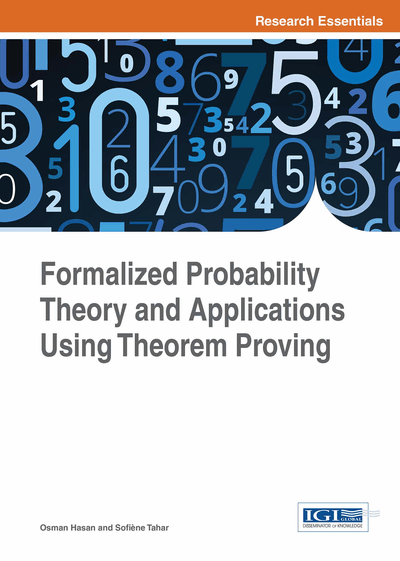# Scheduling Algorithm for Wireless Sensor Networks

DOI: 10.4018/978-1-4666-8315-0.ch013
Available
\$37.50
No Current Special Offers

## Abstract

In Wireless Sensor Networks (WSNs), scheduling of the sensors is considered to be the most effective energy conservation mechanism. The random and unpredictable deployment of sensors in many WSNs in open fields makes the sensor-scheduling problem very challenging and thus randomized scheduling algorithms are used. The performance of these algorithms is usually analyzed using simulation techniques, which do not offer 100% accurate results. Moreover, probabilistic model checking, when used, does not include a strong support to reason accurately about statistical quantities like expectation and variance. In this chapter, the authors overcome these limitations by using higher-order-logic theorem proving to formally analyze the coverage-based random scheduling algorithm for WSNs. Using the probability theory formalization, described in Chapter 5, the authors formally reason about the probability of some events that are interesting in the context of WSN coverage.
Chapter Preview
Top

## 13.2 Formal Analysis Of The K-Set Randomized Scheduling

The formal probabilistic analysis of any system can be broadly divided into two main steps, i.e., the formalization of the given system, while modeling its random components by the formalized random variables, and using this model to formally verify properties of interest as higher-order-logic theorems. In this section, we develop a HOL4 formalization of the coverage-based random scheduling algorithm for WSNs (Elleuch, Hasan, Tahar & Abid, 2011; Elleuch, Hasan, Tahar & Abid, 2013).

## Complete Chapter List

Search this Book:
Reset